Next: Multi-Time Step Nodal Subcycling Up: Multi-Time Step Subcycling Results Previous: Equal Subcycled to Non-Subcycled   Contents

## Unequal Subcycled to Non-Subcycled Region Ratio

Over the next two problems, we increase the region ratio to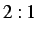( having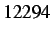nodes,edges, and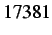volumetric elements ) and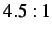( havingnodes,edges, and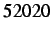volumetric elements ). We plot the displacement profiles of a random node for each of the region ratios in Figures 4.3 and 4.4, respectively. The corresponding timing results are presented in Tables 4.2 and 4.3. For theregion ratio case, the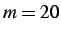result is not presented because the solution became unstable late in the simulation, possibly due to lack of local computer memory.

As we can see from the two figures, the displacements for each of the subcycling parameters are very close to the reference solution; in fact, the differences are nearly imperceptible at certain points in the simulation. In addition, the time savings for both cases increases as the subcycling parameter increases; with the biggest increase occurring for the lower subcycling parameter transitions. For example, for theregion ratio results, the increase fromto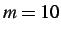generates a savings of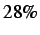, while fromto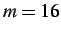it is only. The results for theregion ratio fair slightly better with savings of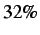and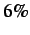, for both subcycling parameter transitions.

Table 4.2: Timing results (in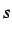) for a problem with an equal region ratio () with subcycling parameters of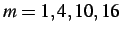and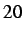.
 Subroutine Reference Case [s], m = 1 m = 4 m = 10 m = 16 m = 20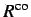438.42 634.97 499.39 487.99 473.49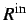1561.98 893.63 483.02 376.92 339.56704.72 994.00 742.12 736.87 700.87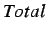2853.85 2693.81 1894.97 1774.19 1682.16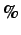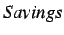6% 34% 38% 41%

Table 4.3: Timing results (in) for a problem with an equal region ratio () with subcycling parameters of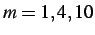and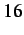.
 Subroutine Reference Case [s], m = 1 m = 4 m = 10 m = 16600.59 886.33 703.14 653.984215.44 2670.78 1264.39 911.161119.75 1115.83 1114.14 1116.317930.09 7577.06 5072.81 4604.524% 36% 42%Next: Multi-Time Step Nodal Subcycling Up: Multi-Time Step Subcycling Results Previous: Equal Subcycled to Non-Subcycled   Contents
Mariusz Zaczek 2002-10-13